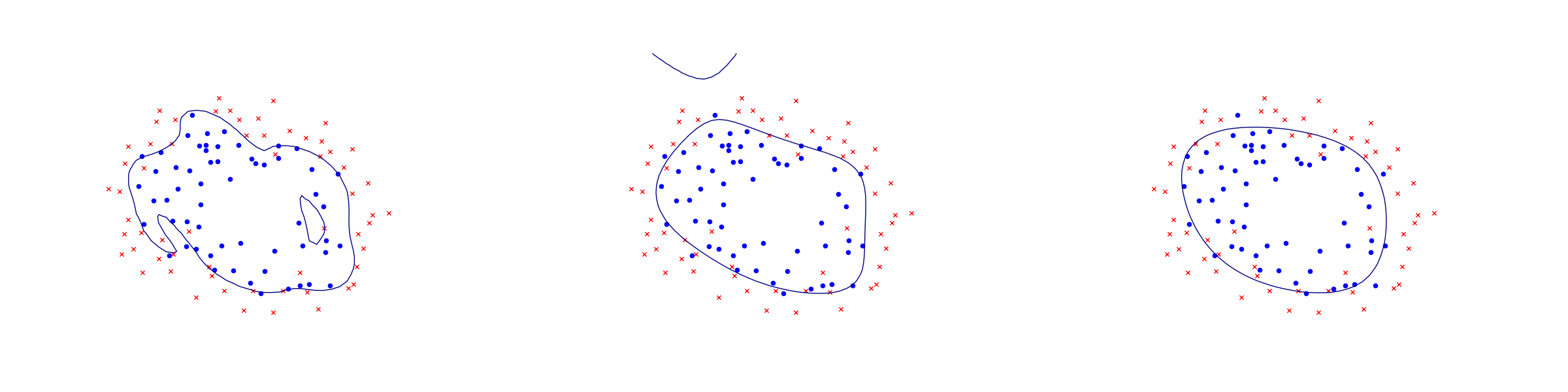# 11.2 Logistic regression¶

Logistic regression is an example of a binary classifier, where the output takes one two values 0 or 1 for each data point. We call the two values classes.

Formulation as an optimization problem

Define the sigmoid function

$S(x)=\frac{1}{1+\exp(-x)}.$

Next, given an observation $$x\in\real^d$$ and a weights $$\theta\in\real^d$$ we set

$h_\theta(x)=S(\theta^Tx)=\frac{1}{1+\exp(-\theta^Tx)}.$

The weights vector $$\theta$$ is part of the setup of the classifier. The expression $$h_\theta(x)$$ is interpreted as the probability that $$x$$ belongs to class 1. When asked to classify $$x$$ the returned answer is

$\begin{split}x\mapsto \begin{cases}\begin{array}{ll}1 & h_\theta(x)\geq 1/2, \\ 0 & h_\theta(x)<1/2.\end{array}\end{cases}\end{split}$

When training a logistic regression algorithm we are given a sequence of training examples $$x_i$$, each labelled with its class $$y_i\in \{0,1\}$$ and we seek to find the weights $$\theta$$ which maximize the likelihood function

$\prod_i h_\theta(x_i)^{y_i}(1-h_\theta(x_i))^{1-y_i}.$

Of course every single $$y_i$$ equals 0 or 1, so just one factor appears in the product for each training data point. By taking logarithms we can define the logistic loss function:

$J(\theta) = -\sum_{i:y_i=1} \log(h_\theta(x_i))-\sum_{i:y_i=0}\log(1-h_\theta(x_i)).$

The training problem with regularization (a standard technique to prevent overfitting) is now equivalent to

$\min_\theta J(\theta) + \lambda\|\theta\|_2.$

This can equivalently be phrased as

(11.13)$\begin{split}\begin{array}{lrllr} \minimize & \sum_i t_i +\lambda r & & & \\ \st & t_i & \geq - \log(h_\theta(x)) & = \log(1+\exp(-\theta^Tx_i)) & \mathrm{if}\ y_i=1, \\ & t_i & \geq - \log(1-h_\theta(x)) & = \log(1+\exp(\theta^Tx_i)) & \mathrm{if}\ y_i=0, \\ & r & \geq \|\theta\|_2. & & \end{array}\end{split}$

Implementation

As can be seen from (11.13) the key point is to implement the softplus bound $$t\geq \log(1+e^u)$$, which is the simplest example of a log-sum-exp constraint for two terms. Here $$t$$ is a scalar variable and $$u$$ will be the affine expression of the form $$\pm \theta^Tx_i$$. This is equivalent to

$\exp(u-t) + \exp(-t)\leq 1$

and further to

(11.14)$\begin{split}\begin{array}{rclr} (z_1, 1, u-t) & \in & \EXP & (z_1\geq \exp(u-t)), \\ (z_2, 1, -t) & \in & \EXP & (z_2\geq \exp(-t)), \\ z_1+z_2 & \leq & 1. & \end{array}\end{split}$

This formulation can be entered using affine conic constraints (see Sec. 6.2 (From Linear to Conic Optimization)).

Listing 11.8 Implementation of $$t\geq \log(1+e^u)$$ as in (11.14). Click here to download.
// Adds ACCs for t_i >= log ( 1 + exp((1-2*y[i]) * theta' * X[i]) )
// Adds auxiliary variables, AFE rows and constraints
MSKrescodee softplus(MSKtask_t task, int d, int n, MSKint32t theta, MSKint32t t, double* X, int* y)
{
MSKint32t nvar, ncon;
MSKint64t nafe, thetaafe, tafe, z1afe, z2afe, oneafe, expdomain;
MSKint32t z1, z2, zcon, v1con, v2con;
MSKint32t  *subi = (MSKint32t*) calloc(2*n, sizeof(MSKint32t));
MSKint32t  *subj = (MSKint32t*) calloc(3*n, sizeof(MSKint32t));
MSKrealt   *aval = (MSKrealt*) calloc(2*n, sizeof(MSKrealt));
MSKint64t  *afeidx = (MSKint64t*) calloc(d*n+4*n, sizeof(MSKint64t));
MSKint32t  *varidx = (MSKint32t*) calloc(d*n+4*n, sizeof(MSKint32t));
MSKrealt   *fval   = (MSKrealt*) calloc(d*n+4*n, sizeof(MSKrealt));
MSKint64t  idx;
int        k, i, j;
MSKrescodee res = MSK_RES_OK;

MSKCALL(MSK_appendcons(task, n));     // z1 + z2 = 1
MSKCALL(MSK_appendafes(task, 4*n));   //theta * X[i] - t[i], -t[i], z1[i], z2[i]

z1 = nvar, z2 = nvar+n;
zcon = ncon;
thetaafe = nafe, tafe = nafe+n, z1afe = nafe+2*n, z2afe = nafe+3*n;

// Linear constraints
k = 0;
for(i = 0; i < n; i++)
{
// z1 + z2 = 1
subi[k] = zcon+i;  subj[k] = z1+i;  aval[k] = 1;  k++;
subi[k] = zcon+i;  subj[k] = z2+i;  aval[k] = 1;  k++;
}
MSKCALL(MSK_putconboundsliceconst(task, zcon, zcon+n, MSK_BK_FX, 1, 1));
MSKCALL(MSK_putvarboundsliceconst(task, nvar, nvar+2*n, MSK_BK_FR, -inf, inf));

// Affine conic expressions
k = 0;

// Thetas
for(i = 0; i < n; i++) {
for(j = 0; j < d; j++) {
afeidx[k] = thetaafe + i; varidx[k] = theta + j;
fval[k] = ((y[i]) ? -1 : 1) * X[i*d+j];
k++;
}
}

// -t[i]
for(i = 0; i < n; i++) {
afeidx[k] = thetaafe + i; varidx[k] = t + i; fval[k] = -1; k++;
afeidx[k] = tafe + i;     varidx[k] = t + i; fval[k] = -1; k++;
}

// z1, z2
for(i = 0; i < n; i++) {
afeidx[k] = z1afe + i; varidx[k] = z1 + i; fval[k] = 1; k++;
afeidx[k] = z2afe + i; varidx[k] = z2 + i; fval[k] = 1; k++;
}

// Add a single row with the constant expression "1.0"

// Add an exponential cone domain

// Conic constraints
for(i = 0; i < n; i++)
{
idx = z1afe+i, idx = oneafe, idx = thetaafe+i;
idx = z2afe+i, idx = oneafe, idx = tafe+i;
}

free(subi); free(subj); free(aval);
free(afeidx); free(varidx); free(fval);
return res;
}


Once we have this subroutine, it is easy to implement a function that builds the regularized loss function model (11.13).

Listing 11.9 Implementation of (11.13). Click here to download.
// Model logistic regression (regularized with full 2-norm of theta)
// X - n x d matrix of data points
// y - length n vector classifying training points
// lamb - regularization parameter
MSKrescodee logisticRegression(MSKenv_t       env,
int            n,    // num samples
int            d,    // dimension
double        *X,
int           *y,
double         lamb,
double        *thetaVal)   // result
{
MSKrescodee res = MSK_RES_OK;
MSKrescodee trm = MSK_RES_OK;
MSKint32t nvar = 1+d+n;
MSKint32t r = 0, theta = 1, t = 1+d;
int i = 0;

// Variables [r; theta; t]
MSKCALL(MSK_putvarboundsliceconst(task, 0, nvar, MSK_BK_FR, -inf, inf));

// Objective lambda*r + sum(t)
for(i = 0; i < n && res == MSK_RES_OK; i++)

// Softplus function constraints
MSKCALL(softplus(task, d, n, theta, t, X, y));

// Regularization
// Append a sequence of linear expressions (r, theta) to F
for(i = 0; i < d; i++)
MSKCALL(MSK_putafefentry(task, numafe + i + 1, theta + i, 1.0));

// Solution

In the next figure we apply logistic regression to the training set of 2D points taken from the example ex2data2.txt . The two-dimensional dataset was converted into a feature vector $$x\in\real^{28}$$ using monomial coordinates of degrees at most 6.Fig. 11.2 Logistic regression example with none, medium and strong regularization (small, medium, large $$\lambda$$). Without regularization we get obvious overfitting.¶